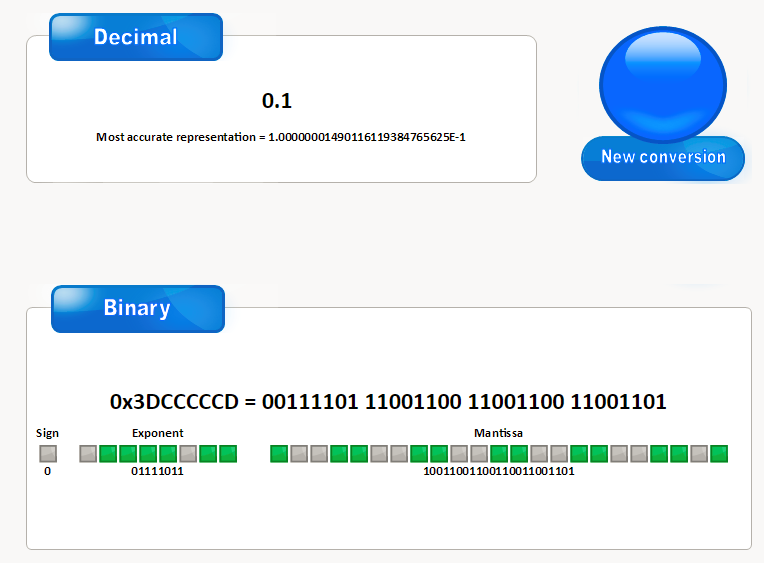# 浮点数的二进制表示以及几个例子 #130

Open
opened this issue Dec 3, 2017 · 0 comments
Open

# 浮点数的二进制表示以及几个例子#130

opened this issue Dec 3, 2017 · 0 comments
Labels

###zhangyachen commented Dec 3, 2017 • edited

int x = 0.58 * 100;
printf("%d",x);        //57,not 58
printf("%d",0.1 + 0.2 == 0.3);        //0,not 1
printf("%f\n",3.14f + 1e10f - 1e10f);            //0.0,not 3.14
printf("%f\n",3.14f + (1e10f - 1e10f));         //3.14
float d1, d2, d3, d4;

d1 = 194268.02f;
d2 = 194268f;
d4 = 0.02f;

d3 = d1 - d2;
if (d3 > d4)
printf(">0.02\n");
else if (d3 < d4)
printf("<0.02\n");     //true
else
printf("=0.02\n");     //false

printf("%f - %f = %f \n", d1,d2,d3);   //194268.015625 - 194268.000000 = 0.015625,not 194268.02 - 194268 = 0.02

double d1, d2, d3, d4;

d1 = 194268.02;
d2 = 194268;
d4 = 0.02;

d3 = d1 - d2;
if (d3 > d4)
printf(">0.02\n");
else if (d3 < d4)
printf("<0.02\n");       //true
else
printf("=0.02\n");      //false

printf("%f - %f = %f \n", d1,d2,d3);    //194268.020000 - 194268.000000 = 0.020000 
gcc compare.c -o compare.o
float p3x = 80838.0f;
float p2y = -2499.0f;
double v321 = p3x * p2y;
printf("%f",v321);          //-202014160,not -202014162

gcc编译时加上-mfpmath选项：

gcc -mfpmath=387 compare.c -o compare.o
float p3x = 80838.0f;
float p2y = -2499.0f;
double v321 = p3x * p2y;
printf("%f",v321);          //-202014162,not -202014160

test.c

float x=1.1;
float z=1.123;
float y=x;
for(int j=0;j<90000000;j++)
{
y*=x;
y/=z;
y+=0.1f;
y-=0.1f;
}

test1.c

float x=1.1;
float z=1.123;
float y=x;
for(int j=0;j<90000000;j++)
{
y*=x;
y/=z;
y+=0;        //diffenent
y-=0;        //different
}

time ./test.o

real	0m1.520s
user	0m1.520s
sys	0m0.001s

time ./test1.o                      //slower about 10 times

real	0m11.241s
user	0m11.243s
sys	0m0.001s


### 小数的二进制表示0.828125 0.110101 1
0.65625 0.10101 1
0.3125 0.0101 0
0.625 0.101 1
0.25 0.01 0
0.5 0.1 1

0.828125的二进制表示就是0.110101.

### IEEE浮点数表示• (-1)^s表示符号位
• IEEE规定M表示有效数字，大于等于1，小于2。也就是我们上面说的1.0101。但是IEEE默认M的第一位是1，所以把1舍去，在计算机中只存储0101，等到读出来时再加上1。这样可以挤出1位有效位。
• 2^E表示指数位。但是这个E是个无符号整数。所以，E的真实值必须减去一个真实值，8位减去127,11位减去1023。（可以想下为什么是127和1023？）。对于上面的例子，2^E表示为2^129，即10000001。当然E还有特殊情况，我们后面再说。### 浮点数的舍入

IEEE规定了4种舍入操作 :

• 默认的方法是找到最近的匹配（向偶数舍入）。比如1.6舍入到2,1.4舍入到1，这是找到最近的匹配。但是1.5（1 和3的中间数）舍入到哪呢？答案是向偶数舍入，即2。
• 向0舍入。即舍入后的数字要向0靠拢。1.4/1.6/1.5 -> 0，-1.5 -> -1。（C语言中float/double向int转换使用此方式）。
• 向下舍入。即舍入后的数字比舍入前的数字小。 1.4/1.6/1.5 -> 1，-1.5 -> -2。
• 向上舍入。即舍入后的数字比舍入前的数字大。 1.4/1.6/1.5 -> 2，-1.5 -> --1。

### C语言中int/float/double的相互转换

C语言中的float和double在支持IEEE浮点数的机器上对应单精度和双精度浮点数。当int/float/double互相转换时遵循以下的原则（int假设32位，float/double所占字节数在16/32/64位平台上都是32/64位）：

• int转为float不会溢出，可能会被舍入。（因为同为32位，但是float只有23位表示有效数字，8位表示指数，1位表示符号位）。
• int转为double，可以保留精确的数值。（因为double有52位表示有效数字）。
• float转为double，可以保留精确的数值。
• double转为float，因为指数和有效数字位数都变小。所以可能溢出到+∞或者-∞。也可能被舍入。
• double/float转为int，会按照向0舍入的原则。1.999 -> 1.-1.9999 -> -1。